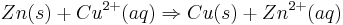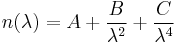# Battery Basics

#### Exploring the Battery

A battery, at its most basic level, is a device that converts chemical energy to electrical energy with an anode, cathode and electrolyte. An anode is the negative electrode, while the cathode is the positive electrode. When immersed in the same electrolyte, the difference in electron concentration creates an electrical potential difference which is utilized for voltage. Certain batteries can be "recharged" by reversing this flow of electrons. The classification of primary batteries include batteries that can not be recharged while secondary batteries can be recharged.Here is an example of a simple galvanic cell with copper and zinc in sulfuric acid. Zinc easily reacts as a positive ion to form zinc sulfate but leaves electrons on the zinc plate. Because copper is less reactive, the positive hydrogen ions deposit on the copper plate. The electrons from the zinc plate then pass through an external conductor to combine with the hydrogen ions on the copper plate until the potential decreases enough.

2000px-Galvanic_Cell.svg.png

The overall reaction for this battery is as follows:$Zn(s) + Cu^{2+}(aq)\Rightarrow Cu(s)+ Zn^{2+}(aq) \,\!$$n(\lambda) = A + \frac {B} {\lambda^2} + \frac {C} {\lambda^4}\,\!$

Together, these half reactions form an oxidation reduction reaction where the Zn is being oxidized, or losing electrons, and the Cu^{2+} is being reduced, or gaining electrons. The potential difference between these two half reactions is the overpotential, which is directly related to voltage efficiency. Voltage is the difference in electrical potential energy between two points of electric charge and has units of volts(V) or Joules/Coulomb(J/C).

#### Battery Specifications

A battery can consist of a cells, modules or packs. A Cell is the smallest, packaged form a battery can take and usually in between 1-6 volts. A module is a series of cells while a pack is a series of connected modules. The flow of electrons (or flow of current) is facilitated by the salt bridge in this case. The rate of discharging this current normalized against the battery capacity is the C-rate as is used to compare different types of batteries. For instance, a 1C rate means the discharge current will discharge the entire battery in 1 hour. E-rates is the discharge power required to discharge the entire battery in 1 hour. Power is the rate at which electrical energy is transferred by an electrical circuit and has units of watt(W) or Joules/Second (J/s). In order to compare battery specifications, the idea of specific power was created. Specific power(W/kg) is a power to weight ratio or the maximum power available per unit of mass and this determines the battery weight required for a performance target. It's counterpart, power density (W/L), is the maximum power per unit volume and is also a characteristic of battery chemistry and packaging. Specific energy (Wh/kg), is the nominal battery energy per unit mass while energy density is the energy per unit volume. While energy measures the total quantity of work being done, power describes how fast that work is being done. Batteries can be classified as high-energy or high power, but never both. Another common classification is high durability which focuses on higher battery life rather than power or energy.

#### Battery Conditions

When analyzing the conditions of a battery, the current conditions are often compared with the maximum or optimal conditions. State of Charge (SOC) (%) is the battery capacity as a percentage of maximum capacity to show the change of capacity over time while Depth of Discharge (DOD)(%) looks at the discharged percent of the capacity maximum capacity. These percentages are important in determining various technical specifications of the battery. For instance, internal resistance depends on the SOC. As the internal resistance increases, the battery efficiency decreases and becomes less stable as the charging energy is converted into heat. Terminal Voltage (V) and Open-circuit voltage (V) both also depend on SOC and DOD. Terminal Voltage is the voltage between battery terminals when a load is applied and Open-Circuit voltage is without a load applied.

#### Department of Energy(DOE) Targets

As renewable energy continues to grow meet a larger percent of our energy needs, battery R&D needs to also continue creating more efficient storage. In the past five years, research funded by the DOE has reduced the cost of plug-in electric vehicle (PEV) batteries by 70% and doubled their energy densities. The DOE's current goal is to reduce the cost of (PEV) batteries to \$125/kWh by 2022 in order to make electric vehicles more competitive with fossil fuel vehicles. Image

#### Explore

PV Education Battery

MIT Electric Battery Team- Guide to Battery Specifications

DOE Vehicle Battery Program